Subsections

6.1 BinaryTree: A Basic Binary Tree

The simplest way to represent a node,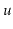, in a binary tree is to explicitly store the (at most three) neighbours of. When one of these three neighbours is not present, we set it to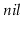. In this way, both external nodes of the tree and the parent of the root correspond to the value.

The binary tree itself can then be represented by a reference to its root node,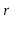: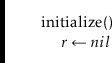We can compute the depth of a node,, in a binary tree by counting the number of steps on the path fromto the root: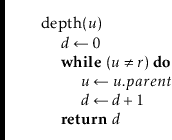6.1.1 Recursive Algorithms

Using recursive algorithms makes it very easy to compute facts about binary trees. For example, to compute the size of (number of nodes in) a binary tree rooted at node, we recursively compute the sizes of the two subtrees rooted at the children of, sum up these sizes, and add one: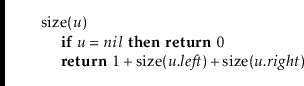To compute the height of a node, we can compute the height of's two subtrees, take the maximum, and add one: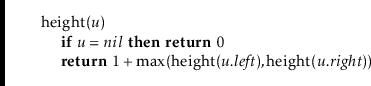6.1.2 Traversing Binary Trees

The two algorithms from the previous section both use recursion to visit all the nodes in a binary tree. Each of them visits the nodes of the binary tree in the same order as the following code: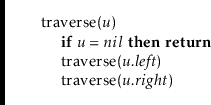Using recursion this way produces very short, simple code, but it can also be problematic. The maximum depth of the recursion is given by the maximum depth of a node in the binary tree, i.e., the tree's height. If the height of the tree is very large, then this recursion could very well use more stack space than is available, causing a crash.

To traverse a binary tree without recursion, you can use an algorithm that relies on where it came from to determine where it will go next. See Figure 6.3. If we arrive at a nodefrom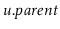, then the next thing to do is to visit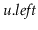. If we arrive atfrom, then the next thing to do is to visit. If we arrive atfrom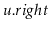, then we are done visiting's subtree, and so we return to. The following code implements this idea, with code included for handling the cases where any of,, oris: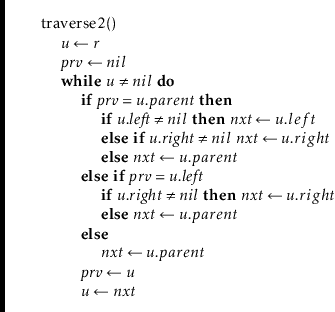Figure 6.3: The three cases that occur at nodewhen traversing a binary tree non-recursively, and the resultant traversal of the tree.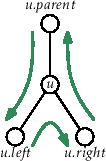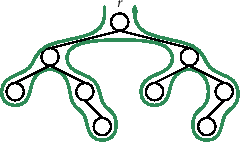The same facts that can be computed with recursive algorithms can also be computed in this way, without recursion. For example, to compute the size of the tree we keep a counter,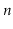, and incrementwhenever visiting a node for the first time: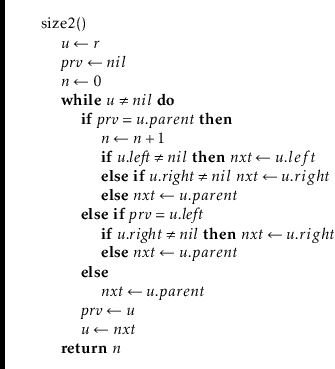In some implementations of binary trees, thefield is not used. When this is the case, a non-recursive implementation is still possible, but the implementation has to use a List (or Stack) to keep track of the path from the current node to the root.

A special kind of traversal that does not fit the pattern of the above functions is the breadth-first traversal. In a breadth-first traversal, the nodes are visited level-by-level starting at the root and moving down, visiting the nodes at each level from left to right (see Figure 6.4). This is similar to the way that we would read a page of English text. Breadth-first traversal is implemented using a queue,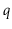, that initially contains only the root,. At each step, we extract the next node,, from, processand addand(if they are non-) to: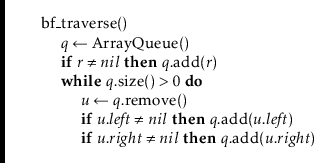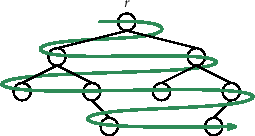opendatastructures.org